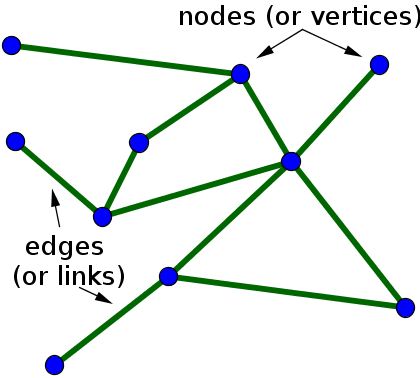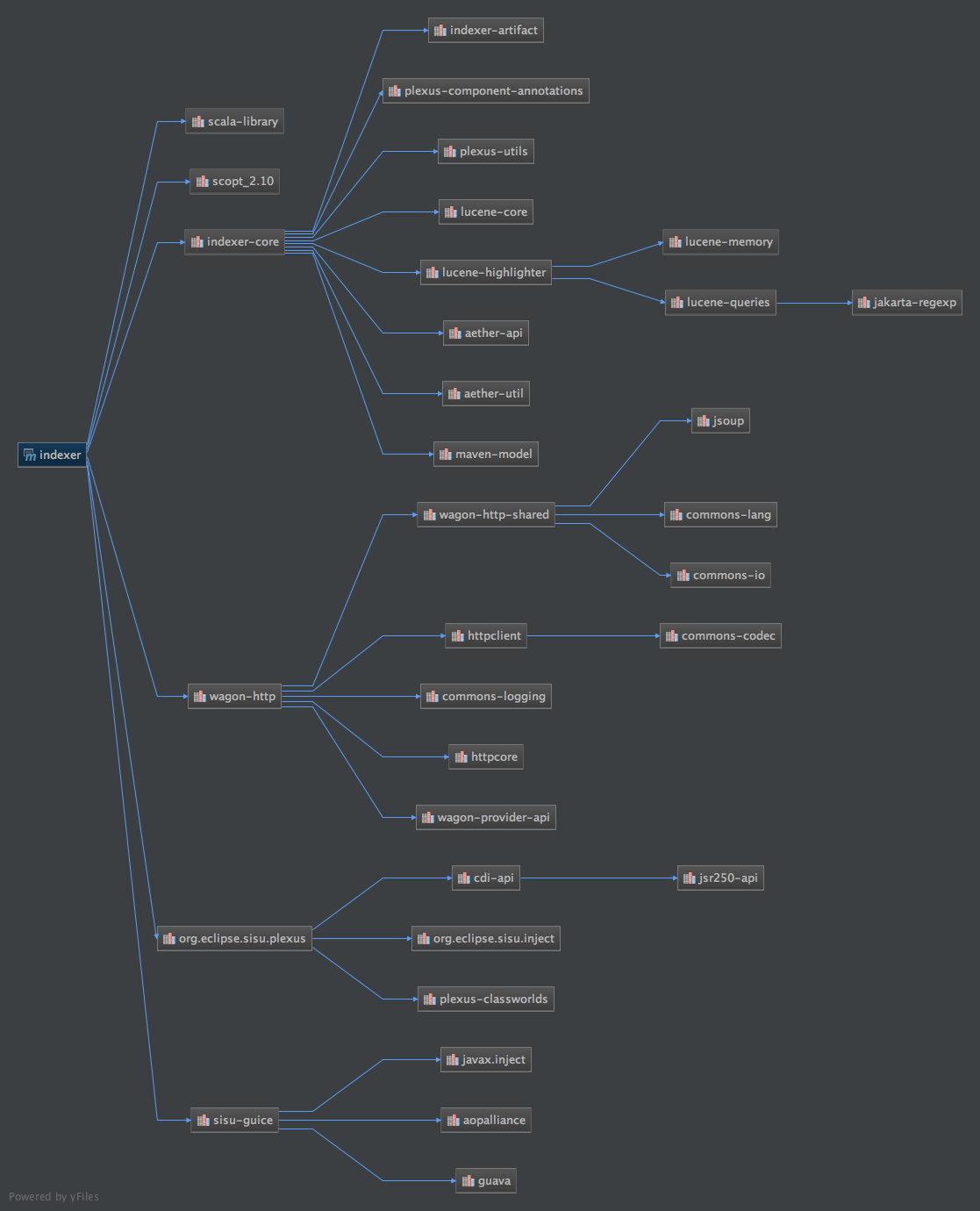# Maven Artifact graph

This post is part of a multipart series about creating a graph off all available Maven dependencies.

In the article for the Neo4j extension I described the input for the extension. This article describes the model that is used to create the required JSon. I start of with a small introduction to Vertices and edges before going into the implementation.

## Vertices and Edges

In mathematics, and more specifically in graph theory, a vertex (plural vertices) or node is the fundamental unit of which graphs are formed: an undirected graph consists of a set of vertices and a set of edges (unordered pairs of vertices), while a directed graph consists of a set of vertices and a set of arcs (ordered pairs of vertices). In a diagram of a graph, a vertex is usually represented by a circle with a label, and an edge is represented by a line or arrow extending from one vertex to another.

From the point of view of graph theory, vertices are treated as featureless and indivisible objects, although they may have additional structure depending on the application from which the graph arises; for instance, a semantic network is a graph in which the vertices represent concepts or classes of objects.

The two vertices forming an edge are said to be the endpoints of this edge, and the edge is said to be incident to the vertices. A vertex w is said to be adjacent to another vertex v if the graph contains an edge (v,w). The neighborhood of a vertex v is an induced subgraph of the graph, formed by all vertices adjacent to v.

Source Wikipedia

The quote above may look is mathmatical definition of a graph. If you translate that text into an image (see below) it is quite clear what is described. A graph consists of nodes and edges. When translated to a Maven dependency graph we replace the nodes for artifacts and the relation between the artifacts as edges.## A Maven graph

The image below depicts a small Maven dependency graph that is part of the application developed. and generated by IntelliJ## Java implementation

In order to interchange the Graph data between Spark and Neo4 we created a domain model that reflects a Graph.

#### Edges

The edges are the simplest to implement, a reference to two vertices is all that needs to be implemented. Issue with the edges in a Maven graph is that it contains more information than just the dependency, Maven has scopes for dependencies, this is added as the third attribute to the edge.

In Source code it looks like this:

``````public class ArtifactEdge implements Serializable {
private final int source;
private final int destination;
private Scope scope;

public ArtifactEdge(final ArtifactVertex source, final ArtifactVertex destination, final Scope scope) {
this.source = source.hashCode();
this.destination = destination.hashCode();
this.scope = scope;
}

public int getSource() {
return source;
}

public int getDestination() {
return destination;
}

public Scope getScope() {
return scope;
}
}
``````

#### Vertices

The vertices reflect the maven artifacts. A artifact is represented by a so called GAV. > Maven GAV’s

a GAV is the maven way of describing an artifact in a unique way and stands for GroupId, ArtifactId and Version.

Although a GAV represents an artifact uniquely still some information is missing. The classifier and packaging is not present in this notation. Commonly used packagings are, jar, war, ear but are not limited to this set. Classifiers are for instance widely used by the Spring team, i.e. the dependency:

``````<dependency>
<groupId>org.springframework</groupId>
<artifactId>spring-core</artifactId>
<version>4.2.5.RELEASE</version>
</dependency>
``````

has the following GAV, classifier and packaging attributes:

• GroupId: org.springframework
• ArtifactId: spring-core
• Version: 4.2.5
• Classifier: RELEASE
• Packaging: jar

For the creation of a correct graph we wanted all this information to be present. Resulting in a class like the following.

``````public class ArtifactVertex implements Serializable {
private final int id;
private final String groupId;
private final String artifactId;
private String version;
private String classifier;
private ArtifactPackaging packaging;

//Constructors, getters, setters and utility methods omitted.
}
``````

#### Graph

Now that the base components are available we can start creating a Grapg. Our representation of the graph is nothing more than two sets, one for the Vertices and one for all the Edges. To connect the Vertices by means of the Edges we added a id field to the Vertices. This allowed the Edge class to be simple. As a result the Graph class is simple and straightforward.

``````public class DependencyGraph implements GSonConverter, Serializable {
private Set<ArtifactVertex> vertices = new HashSet<>();
private List<ArtifactEdge> edges = new ArrayList<>();

//Utility methods omitted
}
``````

## Implementation

The implementation of the model can be found in the Github repository

# Elucidator

Thoughts, stories, How to's and Ideas

© 2013-2019 Pieter van der Meer

Nederburg Hugo Theme by Appernetic.